# 3.10 Comparison of Transport Models

Established techniques used to address dissipative quantum transport can be classified according to the state functions they are based upon: the non-equilibrium GREEN's function (NEGF), the density matrix, and the WIGNER function. All three approaches are based on fundamental equations of motion and are equivalent at the most general level of formal description of a dissipative quantum system.

The resulting system of integral-differential equations for the GREEN's function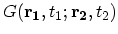, or the density matrix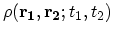, or the WIGNER function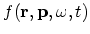would in many cases too complex to allow for a direct numerical solution. For example, the lesser GREEN's function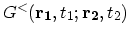in the coordinate representation depends on two positions arguments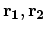and two time arguments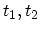. For a numerical solution, each argument of the GREEN's function needs to be discretized. In the case of a three dimensional system the total number of unknowns to be evaluated would be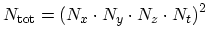. Assuming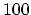grid points for each argument this results in the astronomical number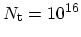. Even in the two-dimensional case the number of unknowns is still very large,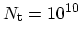, resulting in prohibitively large memory requirement.

Approximations and simplifications must necessarily be incorporated in order to make the problem numerically tractable. It is mainly these simplifying assumptions that make the difference between the approaches. The assumptions are usually physically motivated and may be different in the different formalisms. For instance, the approximations to simplify the equations for the GREEN's functions in real-space may not be suitable to the WIGNER equation, and vice versa.

The hierarchy of the transport models is shown in Fig. 3.11. In what follows we briefly outline strong points and shortcomings of techniques based on the GREEN's function, the density matrix, and the WIGNER function.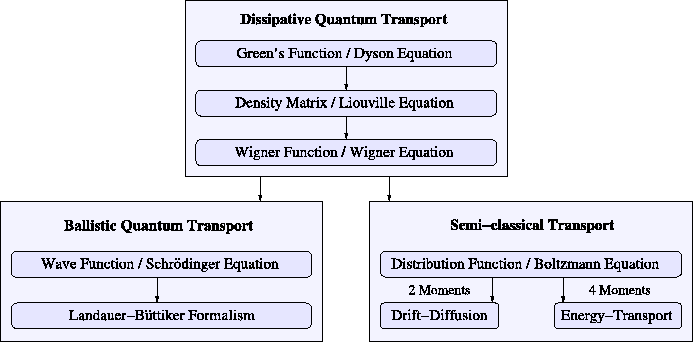Subsections

M. Pourfath: Numerical Study of Quantum Transport in Carbon Nanotube-Based Transistors